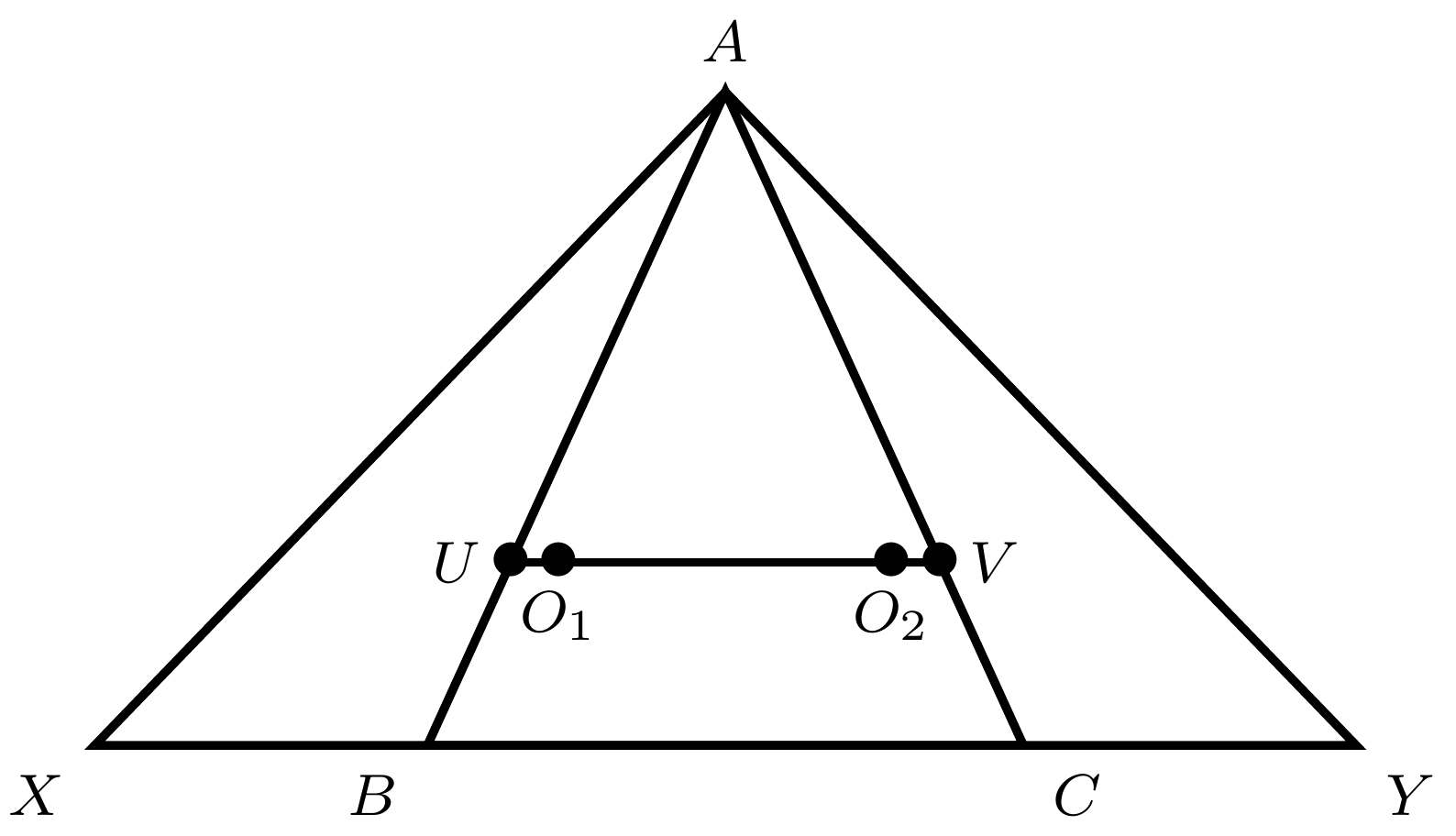###### back to index | new

As shown, two vertices of a square are on the circle and one side is tangent to the circle. If the side length of the square is 8, find the radius of the circle.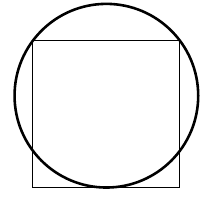$DEB$ is a chord of a circle such that $DE=3$ and $EB=5 .$ Let $O$ be the center of the circle. Join $OE$ and extend $OE$ to cut the circle at $C.$ Given $EC=1,$ find the radius of the circle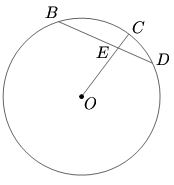Chords $AB$ and $CD$ of a given circle are perpendicular to each other and intersect at a right angle at point $E$. If $BE=16$, $DE=4$, and $AD=5$, find $CE$.

Let $O_1$ and $O_2$ be two intersecting circles. Let a common tangent to these two circles touch $O_1$ at $A$ and $O_2$ at $B$. Show that the common chord of these two circles, when extended, bisects segment $AB$.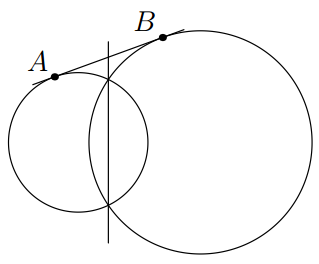Line $PT$ is tangent to circle $O$ at point $T$. $PA$ intersects circle $O$ and its diameter $CT$ at $B$, $D$, and $A$ in that order. If $CD=2, AD=3,$ and $BD=6$, find the length of $PB$.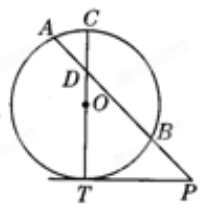As shown, points $X$ and $Y$ are on the extension of $BC$ in $\triangle{ABC}$ such that the order of these four points are $X$, $B$, $C$, and $Y$. Meanwhile, they satisfy the relation $BX\cdot AC = CY\cdot AB$. Let $O_1$ and $O_2$ be the circumcenters of $\triangle{ACX}$ and $\triangle{ABY}$, respectively. If $O_1O_2$ intersects $AB$ and $AC$ at $U$ and $V$, respectively, show that $\triangle{AUV}$ is isosceles.